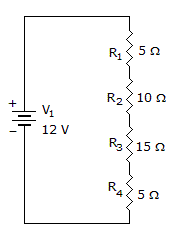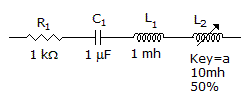# Electronics - Series Circuits

### Exercise :: Series Circuits - True or False

1.

The total resistance of a series circuit is equal to the average of all the resistance values.

 A. True B. False

Answer: Option B

Explanation:

No answer description available for this question. Let us discuss.

2.

The total resistance of a series circuit always depends on the highest value resistor in that circuit.

 A. True B. False

Answer: Option B

Explanation:

No answer description available for this question. Let us discuss.

3.If four 9 V batteries are connected series-aiding, the total voltage is 36 V.

 A. True B. False

Answer: Option A

Explanation:

No answer description available for this question. Let us discuss.

4.

The total resistance is 35.A. True B. False

Answer: Option A

Explanation:

No answer description available for this question. Let us discuss.

5.

These components are in series.A. True B. False

Answer: Option A

Explanation:

No answer description available for this question. Let us discuss.

#### Current Affairs 2021

Interview Questions and Answers